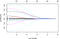# Ridge & Lasso Regression

## Solving overfitting and underfitting problems of the linear regression by using some new regression techniques.

We already learned Linear Regression, so what’s new here? What was the need for Ridge & Lasso Regression technique? We will be answering all these questions right here in detail. First: let’s look at possible categories of results that can be obtained from training a model with linear regression.

Underfitting occurs when the model doesn’t work well with both training data and testing data (meaning the accuracy of both training & testing datasets is below 50%). A possible solution is applying Data Wrangling (data preprocessing or feature engineering).

A model is a Good Fit when it works well with both training and testing datasets (meaning the accuracy for both datasets is around 70%–85% in general cases). It means that we almost achieved our goal.

Overfitting occurs when the model works very well with the training dataset, but on the testing dataset, it fails (meaning that the accuracy of training data is >90% and testing data is < 65%). Since the model works best only on training data and whenever it faces a new situation during testing, it gives wrong results. It is also called a model with high variance. A possible solution is to use the correct regression technique, that is Ridge or Lasso.

# How Ridge Regression Works? How it solves Overfitting?

Ridge regression adds one more term to Linear regression’s cost function. The main reason these penalty terms are added is to make sure there is regularization that is, shrinking the weights of the model to zero or close to zero, to make sure that the model does not overfit the data.

In the context of machine learning, regularization is the process that regularizes or shrinks the coefficients towards zero. In simple words, regularization discourages learning a more complex or flexible model to prevent overfitting. Let’s look at a cost function for a better understanding.

The Residual Sum of Squares measures the amount of error staying between the regression function and the data set. It is a measure of the amount of statistical variance present in the dataset.

For fixed values of lambda in the second term, the multiplication of lambda along with c yields a constant term. So essentially, we will be reducing the equation we have for the ridge above. Lambda (or alpha) is a hyper-parameter that we tune and we set it to a particular value based on our choice. If it is set to zero then the equation of ridge gets converted to that of normal linear regression. The value of lambda will be chosen by cross-validation.

α can take various values:

α = 0:

• The goal becomes the same as simple linear regression.
• We’ll get the same coefficients as simple linear regression.

α = ∞:

• The coefficients will be zero. Why? Because of infinite weightage on a square of coefficients, anything less than zero will make the objective infinite.

0 < α < ∞:

• The magnitude of α will decide the weightage given to different parts of the cost function.
• The coefficients will be somewhere between 0 and ones for simple linear regression.

I hope this gives some sense of how α would impact the magnitude of coefficients. The plot shows cross-validated mean squared error. As lambda decreases, the mean squared error decreases.Ridge includes all the variables in the model and the value of lambda selected is indicated by the vertical lines.

Cross-validation trains the algorithm on a training dataset and then runs the trained algorithm on a validation set. Lambda values are influenced by reducing the percentage of errors of the trained algorithm on the validation set. Overall, choosing a proper value of Lambda for ridge regression allows it to properly fit data in machine learning tasks that use ill-posed problems.

This will eventually reduce overfitting, though our model won’t perform well with training data if the will is more generalized, and also it will give better results on the test dataset.

# Now, overfitting is also solved. So what’s new Lasso does?

Lasso Regression: LASSO stands for Least Absolute Shrinkage and Selection Operator. I know it doesn’t give much of an idea but there are two main keywords here — “absolute and “selection.

The cost function for Lasso (Least Absolute Shrinkage and Selection Operator) regression can be written as:

Thus, from watching the cost function, the only difference found between Ridge & Lasso is that: instead of taking the square of the coefficients, magnitudes are taken into account. This type of regularization can lead to zero coefficients, i.e. some of the features are completely neglected for evaluating output. So Lasso regression not only helps in reducing overfitting but can help us in feature selection. Ridge regression only reduces the coefficients close to zero but not zero, whereas Lasso regression can reduce coefficients of some features to zero, thus resulting in better feature selection. Same as in regression, where also the hyperparameter Lambda can be controlled and all the other functioning works the same here.

Finally, to end this blog, let’s summarize what we have learned so far

1. The cost function of Ridge and Lasso regression and importance of regularization.
2. Hyperparameters reduce the coefficient to zero (or near to zero) to generalize the model.
3. Lasso regression can lead to better feature selection, whereas Ridge can only shrink coefficients close to zero.

NOTE: Based on my experience, Ridge regression performs better than Lasso regression usually for a simpler dataset. Try to use Lasso regression only when there are too many features. That’s all for today, see ya!

Learning and exploring this beautiful world with amazing tech.

## More from Deep Patel

Learning and exploring this beautiful world with amazing tech.